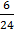### Sample Problem

Alex has 30 dollars. Then he spent 6 dollars on his food.

(a)What is the ratio of the used money to the ratio of the remaining money?

:

(b)What is the ratio of the remaining money to total amount of money?

:

(c)What fraction of the remaining money is the used money?

___

(d)What fraction of the total amount of money is the remaining money?

___

#### Solution

(a) The remaining money is (30 - 6 = 24) dollars. So, ratio of the used money to the ratio of the remaining money is 6:24.

(b) The remaining money is 24 dollars and the total money is 30 dollars. Thus, the ratio is 24:30.

(c) 6:24 andexpress the same relationship between the used money and the remaining money. Thus, the fraction is.

(d) 24:30 andexpress the same relationship between the remaining money and the total money. Thus, the fraction is.